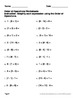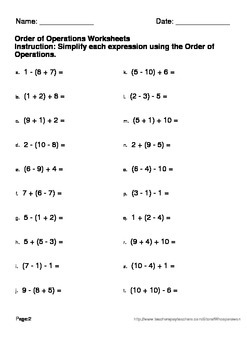## Order of Operations Worksheets\$5.00Buy This Resource Now

Order of Operations:
This package includes 180 worksheets.
Answers included.

Contains negative numbers.

Table of Contents.
1) 3 numbers, addition, subtraction and parentheses. (30 worksheets)
Example: 3 + (2 - 1) =

2) 4 numbers, addition, subtraction and parentheses. (30 worksheets)
Example: 4 - 4 + (8 - 2) =

3) 3 numbers, addition, subtraction, multiplication and parentheses. (30 worksheets)
Example: (2 x 2) - 1 =

4) 4 numbers, addition, subtraction, multiplication and parentheses. (30 worksheets)
Example: 4 - (4 x 1 + 2) =

5) 3 numbers, addition, subtraction, multiplication, division and parentheses. (30 worksheets)
Example: (4 ÷ 2) + 3 =

6) 4 numbers, addition, subtraction, multiplication, division and parentheses. (30 worksheets)
Example: (9 ÷ 3 + 6) x 1 =

----------------------------------

You May Also Like These Task Cards:
Order of Operations Task Cards

Be the first to know about my new discounts, freebies and product launches:
- Look for the green star next to my store logo and click it to become a follower. You will now receive email updates about this store.
- Follow me on Facebook
- Follow me on Pinterest

File Type:
PDF (Acrobat) Document File
Be sure that you have an application to open this file type before downloading and/or purchasing.
200 pages